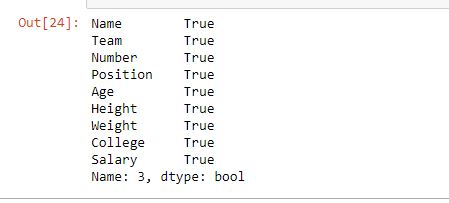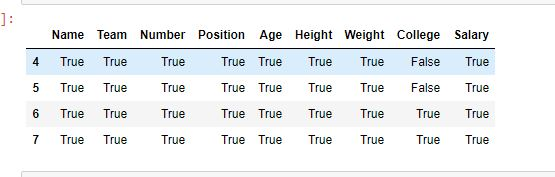Skip to content
Related Articles
Python | Extracting rows using Pandas .iloc[]
• Difficulty Level : Medium
• Last Updated : 17 Sep, 2018

Python is a great language for doing data analysis, primarily because of the fantastic ecosystem of data-centric Python packages. Pandas is one of those packages and makes importing and analyzing data much easier.

Pandas provide a unique method to retrieve rows from a Data frame. `Dataframe.iloc[]` method is used when the index label of a data frame is something other than numeric series of 0, 1, 2, 3….n or in case the user doesn’t know the index label. Rows can be extracted using an imaginary index position which isn’t visible in the data frame.

Syntax: pandas.DataFrame.iloc[]

Parameters:
Index Position: Index position of rows in integer or list of integer.

Return type: Data frame or Series depending on parameters

To download the CSV used in code, click here.

Example #1: Extracting single row and comparing with .loc[]

In this example, same index number row is extracted by both .iloc[] and.loc[] method and compared. Since the index column by default is numeric, hence the index label will also be integers.

 `# importing pandas package``import` `pandas as pd`` ` `# making data frame from csv file ``data ``=` `pd.read_csv(``"nba.csv"``)`` ` `# retrieving rows by loc method ``row1 ``=` `data.loc[``3``]`` ` `# retrieving rows by iloc method``row2 ``=` `data.iloc[``3``]`` ` `# checking if values are equal``row1 ``=``=` `row2`

Output:
As shown in the output image, the results returned by both the methods are same.Example #2: Extracting multiple rows with index

In this example, multiple rows are extracted first by passing a list and then by passing integers to extract rows between that range. After that, both the values are compared.

 `# importing pandas package``import` `pandas as pd`` ` `# making data frame from csv file ``data ``=` `pd.read_csv(``"nba.csv"``)`` ` `# retrieving rows by loc method ``row1 ``=` `data.iloc[[``4``, ``5``, ``6``, ``7``]]`` ` `# retrieving rows by loc method ``row2 ``=` `data.iloc[``4``:``8``]`` ` `# comparing values``row1 ``=``=` `row2`

Output:
As shown in the output image, the results returned by both the methods are same. All values are True except values in college column since those were NaN values.Attention geek! Strengthen your foundations with the Python Programming Foundation Course and learn the basics.

To begin with, your interview preparations Enhance your Data Structures concepts with the Python DS Course. And to begin with your Machine Learning Journey, join the Machine Learning – Basic Level Course

My Personal Notes arrow_drop_up